# KSEEB Solutions for Class 8 Maths Chapter 11 Congruency of Triangles Ex 11.4

In this chapter, we provide KSEEB SSLC Class 8 Maths Chapter 11 Congruency of Triangles Ex 11.4 for English medium students, Which will very helpful for every student in their exams. Students can download the latest KSEEB SSLC Class 8 Maths Chapter 11 Congruency of Triangles Ex 11.4 pdf, free KSEEB SSLC Class 8 Maths Chapter 11 Congruency of Triangles Ex 11.4 pdf download. Now you will get step by step solution to each question.

### Karnataka State Syllabus Class 8 Maths Chapter 11 Congruency of Triangles Ex 11.4

Question 1.
In the given figure. If AB || DC and P is the midpoint of BD prove that P is also the midpoint of BD prove that p is also the midpoint of AC.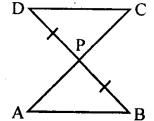In ∆ PDC and ∆ PAB
PD = PB (data)
∠DPC = ∠APB [V.O.A]
∠PD∆ = ∠PBA [Alternate angles AB || CD]
∴ ∆ PDC ≅ ∆ PAB [ASA postulate]
∴ PC = PA [Corresponding sides]
∴ P is the midpoint AC

Question 2.
In the adjacent figure, CD and BE are altitudes of an isosceles triangle ABC with AC = AB prove that AE = AD.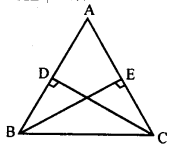AC = AB [data]
∠ADC = ∠AEB[BE & CD altitudes]
∠DAC =∠EAB [Common angle]

Question 3.
In the figure, AP and BQ are perpendiculars to the line segment AB and AP = BQ. Prove that O is the midpoint of line segment AB as well as PQ.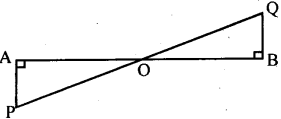In ∆APO and ∆BQO
AP = BQ [data]
∠POA = ∠BOQ [ V.O.A]
∠PAO = ∠QBO = 90°
[AP & BQ are perpendiculars]
∴ ∆APO = ∆BQO [AS∆ postulate]
∴ AO = BO [Corresponding sides]
PO = OQ
∴ O is the midpoint of AB and PQ.

Question 4.
Suppose ABC is an isosceles triangle with AB = AC, BD and CE are bisectors of|fi andlC. Prove that BD = CE.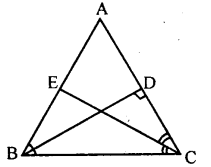Ans.
In ∆ABD and ∆ACE
AB = AC [data]
∠ABD = ∠ACE [∠B =∠C and BD and CE are anglar bisectors]
∴ ∆ ABD ≅ ∆ ACE [AS∆ postulate]
BD = CE [Corresponding sides]

Question 5.
Suppose ABC is an equiangular triangle. Prove that it is equilateral. (You have seen earlier that an equilateral triangle is equiangular. Thus for triangles equiangularity is equivalent is equilaterality.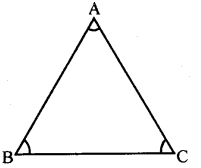ABC is an equiangular triangle i.e.,
∠A =∠B =∠C
∠A = ∠B
BC = AC …(I) [Theorem2]
∠A =∠C
∴BC = AB ….(I) [Theorem2]
From (i) and (ii)
AB = BC = AC
∴ ∆ABC is equilateral.

All Chapter KSEEB Solutions For Class 8 maths

—————————————————————————–

All Subject KSEEB Solutions For Class 8

*************************************************

I think you got complete solutions for this chapter. If You have any queries regarding this chapter, please comment on the below section our subject teacher will answer you. We tried our best to give complete solutions so you got good marks in your exam.

If these solutions have helped you, you can also share kseebsolutionsfor.com to your friends.

Best of Luck!!